# JAC Class 10 Maths Solutions Chapter 6 Triangles Ex 6.6

Jharkhand Board JAC Class 10 Maths Solutions Chapter 6 Triangles Ex 6.6 Textbook Exercise Questions and Answers.

## JAC Board Class 10 Maths Solutions Chapter 6 Triangles Exercise 6.6

Question 1.
In the given figure, PS is the bisector of ∠QPR of ΔPQR. Prove that $$\frac{QS}{SR}=\frac{PQ}{PR}$$
Solution :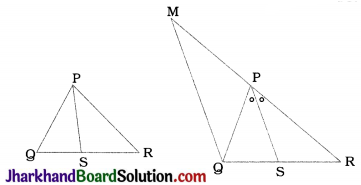Construction: Through Q, draw a line parallel to PS which intersects RP extended at M.
Proof: In ΔMQR, S and P are points on QR and MR respectively and PS || MQ.
∴ $$\frac{QS}{SR}=\frac{MP}{PR}$$ (BPT) ……………(1)
Now, PS || MQ and PQ is their transversal.
∴ ∠SPQ = ∠PQM (Alternate angles) ……(2)
Similarly, PS || MQ and MR is their transversal.
∴ ∠RPS = ∠PMQ (Corresponding angles) …………….(3)
PS is the bisector of ∠QPR.
∴ ∠SPQ = ∠RPS …………….(4)
From (2), (3) and (4).
∠PQM = ∠PMQ.
∴ In ΔPMQ, MP = PQ ……….(5)
From (1) and (5), we get
$$\frac{QS}{SR}=\frac{PQ}{PR}$$Question 2.
In the given figure, D is a point on hypotenuse AC of ΔABC, such that BD ⊥ AC, DM ⊥ BC and DN ⊥ AB. Prove that:
1. DM² = DN . MC
2. DN² = DM . AN
Solution :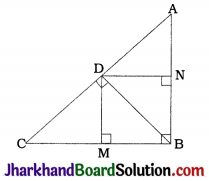In quadrilateral DMBN, ∠B = ∠M = ∠N = 90°.
Hence, DMBN is a rectangle.
∴ DN = MB ………….. (1)
and DM = NB ………….. (2)
Now, BD ⊥ AC
∴ ∠BDC = 90° and ΔBDC is a right triangle in which DM is altitude on hypotenuse BC.
Then, ΔBMD ~ ΔDMC ~ ΔBDC. (Theorem 6.7)
∴ $$\frac{DM}{CM}=\frac{BM}{DM}$$
∴ DM² = BM. CM
∴ DM² = DN. MC [By (1), DN = MB] [Result (1)]
Similarly, ΔADB is a right triangle in which DN is altitude on hypotenuse AB.
∴ ΔAND ~ ΔDNB ~ ΔADB (Theorem 6.7)
∴ $$\frac{DN}{BN}=\frac{AN}{DN}$$
∴ DN2 = BN. AN
∴ DN² = DM . AN [By (2), DM = NB] [Result (2)]

Question 3.
In the given figure, ABC is a triangle in which ∠ABC > 90° and AD ⊥ CB produced. Prove that AC² = AB² + BC² + 2BC . BD.
Solution :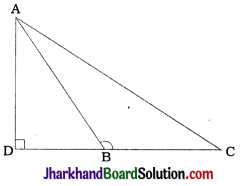∴ AC² = AD² + DC²
∴ AD² = AC² – DC² ……………(1)
∴ AB² = AD² + DB²
∴ AD² = AB² – DB² ……………(2)
From (1) and (2).
AC² – DC² = AB² – DB²
∴ AC² = AB² + DC² – DB²
∴ AC² = AB2 + (BC + DB)² – DB²
∴ AC² = AB² + BC² + 2BC . DB + DB² – DB²
∴ AC² = AB² + BC² + 2BC . BDQuestion 4.
In the given figure, ABC is a triangle in which ∠ABC < 90° and AD ⊥ BC. Prove that AC² = AB² + BC² – 2BC . BD.
Solution :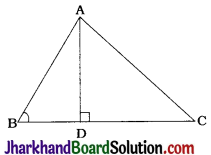∴ AC² = AD² + CD²
∴ AD² = AC² – CD² ……………….(1)
∴ AB² = AD² + BD²
∴ AD² = AB² – BD² ……………….(2)
From (1) and (2),
AC² – CD² = AB² -BD²
∴ AC² = AB² + CD² – BD²
∴ AC² = AB² + (BC – BD)² – BD²
∴ AC² = AB² + BC² – 2BC . BD + BD² – BD²
∴ AC² = AB² + BC² – 2BC . BD

Question 5.
In the given figure, AD is a median of a triangle ABC and AM ⊥ BC. Prove that:
1. AC² = AD² + BC. DM + ($$\frac{BC}{2}$$)²
2. AB² = AD² – BC. DM + ($$\frac{BC}{2}$$)²
3. AC² + AB² = 2AD² + $$\frac{1}{2}$$BC²
Solution :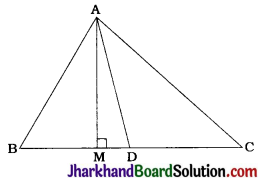Here, ΔAMD, ΔAMC and ΔAMB are right triangles.
Also, since AD is a median, D is the midpoint of BC.
∴ CD = BD = $$\frac{BC}{2}$$
Moreover, DM = CM – CD and DM = BD – BM
1. In ΔAMC, ∠M = 90°
∴ AC² = AM² + CM²
∴ AC² = AM² + (DM + CD)²
∴ AC² = AM² + DM² + 2. DM.CD + CD²
∴ AC² = (AM² + DM²) + (2CD) (DM) + CD²
∴ AC² = AD² + BC.DM + ($$\frac{BC}{2}$$)²
(∵ In ΔAMD, AD² = AM² + DM²)

2. In ΔAMB, ∠M = 90°
∴ AB² = AM² + BM²
∴ AB² = AM² + (BD – DM)²
∴ AB² = AM² + BD² – 2 BD.DM + DM²
∴ AB² = (AM² + DM²) – (2BD) · (DM) + BD²
∴ AB² = AD² – BC.DM + ($$\frac{BC}{2}$$)²
(∵ In ΔAMD, AD² = AM² + DM²)

3. Now, adding the results of part (1) and (2)
AC² + AB² = AD² + BC. DM + ($$\frac{BC}{2}$$)² + AD² – BC . DM + ($$\frac{BC}{2}$$)²
∴ AC² + AB² = 2AD² + 2($$\frac{\mathrm{BC}^2}{4}$$)
∴ AC² + AB² = 2AD² + $$\frac{1}{2}$$BC²
[Note: In this result, if we replace BC by 2BD, we get the famous result know as Apollonius theorem : If AD is a median of ΔABC, then AB² + AC² = 2 (AD² + BD²).]Question 6.
Prove that the sum of the squares of the diagonals of a parallelogram is equal to the sum of the squares of its sides.
Solution :
First of all, we prove Apollonius Theorem.
In ΔABC, let AD be a median and AM be an altitude as shown in the figure.
Then, AB² + AC²
= AM² + BM² + AM² + CM²
= 2AM² + (BD – MD)² + (CD + MD)²
= 2AM² + (BD – MD)² + (BD + MD)² (∵ CD = BD)
= 2AM² + 2BD² + 2MD²
= 2(AM² + MD²) + 2BD²
∴ AB² + AC² = 2(AD² + BD²)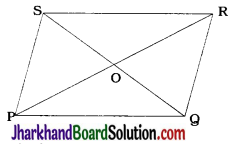Let PQRS be a parallelogram in which the diagonals bisect each other at O.
Then, PO = RO = $$\frac{1}{2}$$PR and
QO = SO = $$\frac{1}{2}$$QS.
Now, in ΔPQR, QO is a median.
∴ PQ² + QR² = 2(QO² + PO²)
∴ PQ² + QR² = 2{($$\frac{QS}{2}$$)² + ($$\frac{PR}{2}$$)²}
∴ PQ² + QR² = $$\frac{1}{2}$$(QS² + PR² ) ………….(1)
Similarly.
in ΔQRS, QR² + RS² = $$\frac{1}{2}$$(QR² + PR²) ……(2)
in ΔRSP, RS² + SP² = $$\frac{1}{2}$$(QS² + PR²) ……….(3)
in ΔSPQ, SP² + PQ² = $$\frac{1}{2}$$(QS² + PR²) ……….(4)
Adding (1), (2), (3) and (4), we get
2(PQ² + QR² + RS² + SP²) = 2(QS² + PR²)
∴ PQ² + QR² + RS² + SP² = QS² + PR²
Thus, the sum of the squares of the diagonals of a parallelogram is equal to the sum of the squares of its sides.

Question 7.
In the given figure, two chords AB and CD intersect each other at the point P. Prove that
1. ΔAPC ~ ΔDPB
2. AP . PB = CP . DP
Solution :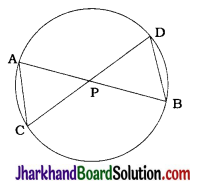Here, ∠CAB = ∠CDB
(Angles in the same segment)
∴ ∠CAP = ∠BDP
Similarly,
∠ACD = ∠DBA
(Angles in the same segment)
∴ ∠ACP = ∠DBP
Now, in ΔAPC and ΔDPB,
∠CAP = ∠BDP and ∠ACP = ∠DBP.
∴ By AA criterion, ΔAPC ~ ΔDPB. (Result 1)
∴ $$\frac{AP}{DP}=\frac{CP}{BP}$$
∴ AP . PB = CP . DP (Result 2)Question 8.
In the given figure, two chords AB and CD of a circle intersect each other at the point P (when produced) outside the circle.
Prove that
1. ΔPAC ~ ΔPDB
2. PA . PB = PC . PD
Solution :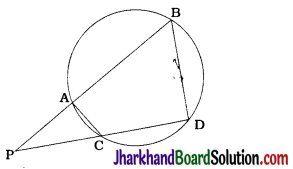ΔACD + ∠ABD = 180°
Again, ∠ACD + ∠ACP = 180° (Linear pair)
∴ ∠ABD = ∠ACP
∴ ∠PBD = ∠PCA.
Similarly, ∠CAB + ∠CDB = 180° (Cyclic quadrilateral)
∠CAB + ∠CAP = 180° (Linear pair)
∴ ∠CDB = ∠CAP
∴ ∠PDB = ∠PAC
Now, in ΔPDB and ΔPAC,
∠PBD = ∠PCA
∠PDB = ∠PAC
∴ By AA criterion, ΔPAC ~ ΔPDB [Result (1)]
∴ $$\frac{PA}{PD}=\frac{PC}{PB}$$
∴ PA.PB = PC.PD [Result (2)]

Question 9.
In the given figure, D is a point on side BC of ΔABC such that $$\frac{BD}{CD}=\frac{AB}{AC}$$. Prove that AD is the bisector of ∠BAC.
Solution :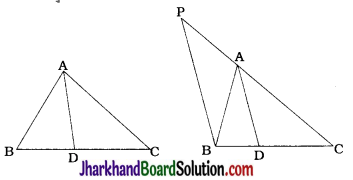Through B. draw a line parallel to AD to intersect CA extended at P.
Then, in ΔPBC, A and D are points on PC and BC respectively and PB || AD.
∴ $$\frac{PA}{AC}=\frac{BD}{CD}$$
Also, $$\frac{BD}{CD}=\frac{AB}{AC}$$ (Given)
∴ PA = AB
Now, in ΔPAB, PA = AB
∴ ∠ABP = ∠APB …………..(1)
AD || BP and AB is their transversal.
∴ ∠ABP = ∠BAD (Alternate angles) …………..(2)
AD || BP and CP is their transversal.
∴ ∠APB = ∠CAD (Corresponding angles) …………..(3)
From (1), (2) and (3),
Hence, AD is the bisector of ∠BAC.Question 10.
Nazima is fly fishing in a stream. The tip of her fishing rod is 1.8 m above the surface of the water and the fly at the end of the string rests on the water 3.6 m away from her and 2.4 m from a point directly under the tip of the rod. Assuming that her string (from the tip of her rod to the fly) is taut, how much string does she have out (see the given figure)? If she pulls in the string at the rate of 5 cm per second, what will be the horizontal distance of the fly from her after 12 seconds?
Solution :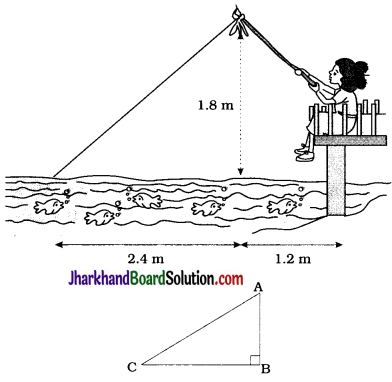Here, ΔABC represents the initial position in which A is the tip of her fishing rod, C is the fly at the end of the string and B is the point directly under the tip of the rod.
Then, in ΔABC, ∠B = 90°, AB = 1.8m and BC = 2.4 m.
∴ AC² = AB² + BC² (Pythagoras theorem)
∴ AC² = (1.8)² + (2.4)²
∴ AC² = 3.24 + 5.76
∴ AC² = 9
∴ AC = 3 m
Hence, in the initial position, she has 3 m of string out.
Length of string pulled-in in 1 sec = 5 cm
∴ Length of string pulled-in in 12 sec = 60 cm = 0.6 m
Now, in the second position, the length of string AC = 3m-0.6 m = 2.4 m and AB = 1.8 m.
Again, AC² = AB² + BC²
∴ (2.4)² = (1.8)² + BC²
∴ BC² = (2.4)² – (1.8)²
∴ BC² = (2.4 + 1.8) (2.4 – 1.8)
∴ BC² = 4.2 × 0.6
∴ BC² = 2.52
∴ BC = $$\sqrt{2.52}$$
∴ BC= 1.59 m (approx)
Now, the horizontal distance of the fly from her
= BC + 1.2 m
= (1.59 + 1.2) m
= 2.79 m